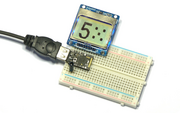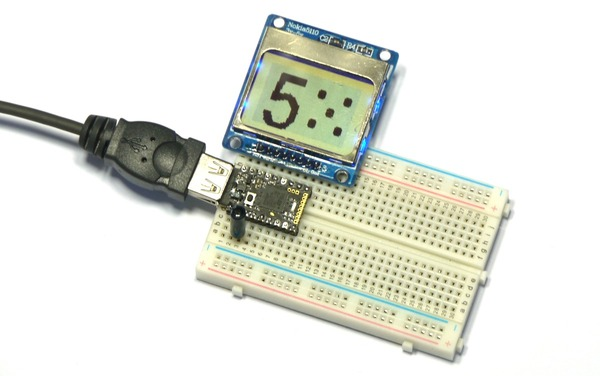Pico Electronic Dice

Introduction

This is a simple electronic dice that comes up with a new random number each time it's shaken.

Wiring UpThis one's really easy...

• Simply place the Espruino Pico on the breadboard with the USB connector facing left
• Put the LCD directly above it, aligned to the right (so the pin on the Pico nearest the USB connector should not be connected to anything)
• Finally push the vibration sensor into the 3rd and 4th holes from the left, on the bottom edge of the Pico board, it can be any way around. Be careful as one of the wires is very delicate and bends easily.

Software

First we'll just copy and paste the code that we used in the Pico Hello World example, with the code from the Pico Vibration example. Put the following in the right-hand side of the Web IDE and click Send to Espruino:

A5.write(0); // GND
A7.write(1); // VCC
A6.write(1); // Turn on the backlight

var g; // Define g globally, so that it can be used by other functions

function onInit() {
// Setup SPI
var spi = new SPI();
spi.setup({ sck:B1, mosi:B10 });
// Initialise the LCD
g = require("PCD8544").connect(spi,B13,B14,B15, function() {
// When it's initialised, clear it and write some text
g.clear();
g.drawString("Hello World!",0,0);
// send the graphics to the display
g.flip();
});
}

var on = false;
function shaken() {
if (on) return; // if we haven't timed out, ignore this
// otherwise, turn the light on
on = true;
digitalWrite(LED1, on);
// now set a timeout to turn it off after 200ms = 1/5 second
setTimeout(function() {
on = false;
digitalWrite(LED1, on);
}, 200);
}

pinMode(B3,"input_pulldown"); // enable the pull-down resistor
// Now 'watch' B3 for shakes
setWatch(shaken, B3, {repeat:true, edge:"rising"});

onInit();

Now, the screen will display "Hello World" and if you shake the board it'll light the LED for a fraction of a second.

The next step is just to modify the shaken function to display a random number - replace it with the following:

function showRandom() {
// get a random number between 1 and 6
var n = 1 + Math.floor(Math.random()*6);
// Clear the screen
g.clear();
// big font - as high as the screen
g.setFontVector(g.getHeight());
// draw the number, in the middle of the screen
g.drawString(n, (g.getWidth()-g.stringWidth(n))/2, 0);
// send to the LCD
g.flip();
}

function shaken() {
if (on) return; // if we haven't timed out, ignore this
// otherwise, turn the light on
on = true;
digitalWrite(LED1, on);
showRandom();
// now set a timeout to turn it off after 200ms = 1/5 second
setTimeout(function() {
on = false;
digitalWrite(LED1, on);
}, 200);
}

And that's it, A simple electronic dice!

You could expand it to draw the dice itself by changing the showRandom function:

// the dots defined on each face
var dots = [
undefined,
[ [0,0] ],
[ [-1,-1],[1,1] ],
[ [1,-1],[0,0],[-1,1] ],
[ [-1,-1],[1,1],[1,-1],[-1,1] ],
[ [-1,-1],[1,1],[1,-1],[-1,1],[0,0] ],
[ [-1,-1],[0,-1],[1,-1],[-1,1],[0,1],[1,1] ],
];

var on = false;
function showRandom() {
// get a random number between 1 and 6
var n = 1 + Math.floor(Math.random()*6);
// Clear the screen
g.clear();
// big font - as high as the screen
g.setFontVector(g.getHeight());
// draw the number
g.drawString(n, (g.getWidth()/2-g.stringWidth(n))/2, 0);
// draw the dice
dots[n].forEach(function(coords) {
var x = g.getWidth()*3/4 + coords*12;
var y = g.getHeight()/2 + coords*12;
g.fillRect(x-3,y-2,x+3,y+2);
g.fillRect(x-2,y-3,x+2,y+3);
});
// send to the LCD
g.flip();
}

This page is auto-generated from GitHub. If you see any mistakes or have suggestions, please let us know.